### Matrix - Sum of elements below and above both diagonal , triangle and more operations on matrix in java

Contents of page >
1) Find sum of elements above diagonal in matrix in java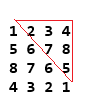2) Find sum of elements below diagonal in matrix in java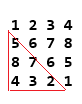3) Find sum of both diagonals in matrix in java4) Find sum of upper triangle in matrix in java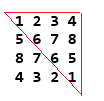5) Find sum of lower triangle in matrix in java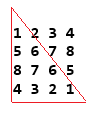6) Display in required format in java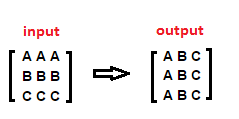7) Matrix Addition in java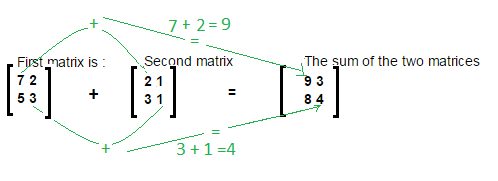8) Matrix Subtraction in java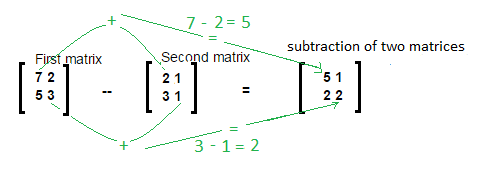9) Matrix Multiplication in java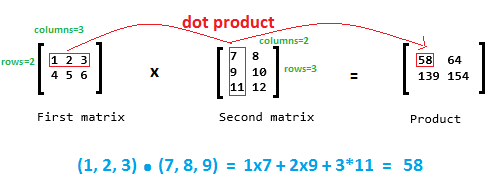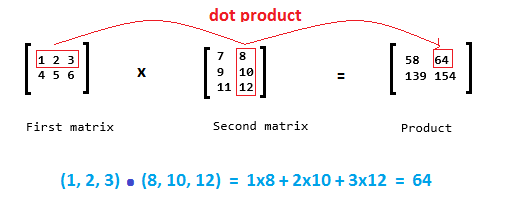10) Matrix Transpose in java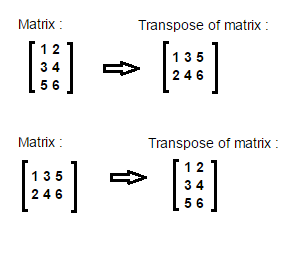1) Find sum of elements above diagonal in matrix in java
Write a program to Find sum of all elements above diagonal in matrix in java.Sum of elements above diagonal = 2+3+4+7+8+5= 29

Logic behind finding sum of elements above diagonal in java is:
//Logic to calculate sum of elements above diagonal.
int sum=0;
for (int j = 1; j < columns; j++) {
for (int i=j-1 ; i>=0 ; i--) {
sum= sum + matrix[i][j];
}

}

Example / Full Program/SourceCode in java >
 package matrix; import java.util.Scanner; /** Copyright (c), AnkitMittal  JavaMadeSoEasy.com */ public class SumOfElementsAboveDiagonal {    public static void main(String...args) {                     Scanner scanner = new Scanner(System.in);           System.out.print("Enter number of rows/columns in matrix : ");    //rows and columns in matrix must be same.           int rows = scanner.nextInt();           int columns=rows;           int[][] matrix = new int[rows][rows];                     System.out.println("Enter the elements in matrix :");           for (int i = 0; i < rows; i++) {                  for (int j = 0; j < columns; j++) {                        matrix[i][j] = scanner.nextInt();                  }           }           //Logic to calculate sum of elements above diagonal.           int sum=0;           for (int j = 1; j < columns; j++) {                  for (int i=j-1 ; i>=0 ; i--) {                        sum= sum + matrix[i][j];                  }                            }           System.out.println("\nMatrix is : ");           for (int i = 0; i < rows; i++) {                  for (int j = 0; j < columns; j++) {                        System.out.print(matrix[i][j] + " ");                  }                  System.out.println();           }           System.out.println("sum of elements above diagonal is: "+sum);    } } /*OUTPUT Enter number of rows/columns in matrix : 4 Enter the elements in matrix : 1 2 3 4 5 6 7 8 8 7 6 5 4 3 2 1 Matrix is : 1 2 3 4 5 6 7 8 8 7 6 5 4 3 2 1 sum of elements above diagonal is: 29   */

2) Find sum of elements below diagonal in matrix in java
Write a program to Find sum of elements below diagonal in matrix in javaSum of elements below diagonal = 5+8+7+4+3+2= 29

Logic behind finding sum of elements below diagonal in java is:
//Logic to calculate sum of elements below diagonal.
int sum=0;
for (int i = 1; i < rows; i++) {
for (int j=i-1 ; j>=0 ; j--) {
sum= sum + matrix[i][j];
}

}

Example / Full Program/SourceCode in java >
 package matrix; import java.util.Scanner; /** Copyright (c), AnkitMittal  JavaMadeSoEasy.com */ public class SumOfElementsBelowDiagonal {    public static void main(String...args) {                     Scanner scanner = new Scanner(System.in);           System.out.print("Enter number of rows/columns in matrix : ");    //rows and columns in matrix must be same.           int rows = scanner.nextInt();           int columns=rows;           int[][] matrix = new int[rows][rows];                     System.out.println("Enter the elements in matrix :");           for (int i = 0; i < rows; i++) {                  for (int j = 0; j < columns; j++) {                        matrix[i][j] = scanner.nextInt();                  }           }                     //Logic to calculate sum of elements below diagonal.           int sum=0;           for (int i = 1; i < rows; i++) {                  for (int j=i-1 ; j>=0 ; j--) {                        sum= sum + matrix[i][j];                  }                            }           System.out.println("\nMatrix is : ");           for (int i = 0; i < rows; i++) {                  for (int j = 0; j < columns; j++) {                        System.out.print(matrix[i][j] + " ");                  }                  System.out.println();           }           System.out.println("sum of elements below diagonal is: "+sum);    } } /*OUTPUT Enter number of rows/columns in matrix : 4 Enter the elements in matrix : 1 2 3 4 5 6 7 8 8 7 6 5 4 3 2 1 Matrix is : 1 2 3 4 5 6 7 8 8 7 6 5 4 3 2 1 sum of elements below diagonal is: 29 */

3) Find sum of both diagonals in matrix in java
Write a program to Find sum of both diagonals in matrix in java.sum of diagonal1 elements= 1+6+6+1=14,
sum of diagonal2 elements= 4+7+7+4=22
sum of diagonal1 and diagonal2 elements = 14+22= 36

Logic behind finding sum of both diagonals in matrix in java is:
//Logic to calculate sum of diagonal1
int sumOfDiagonal1=0;
for (int i = 0, j =0; i< rows && j < columns; i++, j++) {
sumOfDiagonal1= sumOfDiagonal1 + matrix[i][j];

}

//Logic to calculate sum of diagonal2
int sumOfDiagonal2=0;
for (int i=0,j=columns-1 ; i<rows && j>=0 ; i++, j--) {
sumOfDiagonal2= sumOfDiagonal2 + matrix[i][j];

}

Example / Full Program/SourceCode in java >
 package matrix; import java.util.Scanner; /** Copyright (c), AnkitMittal  JavaMadeSoEasy.com */ public class SumOfBothDiagonals {    public static void main(String...args) {                     Scanner scanner = new Scanner(System.in);           System.out.print("Enter number of rows/columns in matrix : ");    //rows and columns in matrix must be same.           int rows = scanner.nextInt();           int columns=rows;           int[][] matrix = new int[rows][rows];                     System.out.println("Enter the elements in matrix :");           for (int i = 0; i < rows; i++) {                  for (int j = 0; j < columns; j++) {                        matrix[i][j] = scanner.nextInt();                  }           }           //Logic to calculate sum of diagonal1           int sumOfDiagonal1=0;           for (int i = 0, j =0; i< rows && j < columns; i++, j++) {                  sumOfDiagonal1= sumOfDiagonal1 + matrix[i][j];                            }                     //Logic to calculate sum of diagonal2           int sumOfDiagonal2=0;           for (int i=0,j=columns-1 ; i=0 ; i++, j--) {                  sumOfDiagonal2= sumOfDiagonal2 + matrix[i][j];                            }           System.out.println("\nMatrix is : ");           for (int i = 0; i < rows; i++) {              for (int j = 0; j < columns; j++) {                        System.out.print(matrix[i][j] + " ");                  }                  System.out.println();           }           System.out.println("sum of diagonal1 elements="+sumOfDiagonal1+", sum of diagonal2 elements ="+ sumOfDiagonal2 );           System.out.println("sum of diagonal1 and diagonal2 elements is: "+ (sumOfDiagonal1+ sumOfDiagonal2) );    } } /*OUTPUT Enter number of rows/columns in matrix : 4 Enter the elements in matrix : 1 2 3 4 5 6 7 8 8 7 6 5 4 3 2 1 Matrix is : 1 2 3 4 5 6 7 8 8 7 6 5 4 3 2 1 sum of diagonal1 elements=14, sum of diagonal2 elements =22 sum of diagonal1 and diagonal2 elements is: 36 */

4) Find sum of upper triangle in matrix in java
Write a program to Find sum of upper triangle in matrix in java.Sum of upper triangle = 1+2+3+4+6+7+8+6+5+1= 43

Logic behind finding sum of upper triangle in matrix in java is:
//Logic to calculate sum of upper triangle.
int sum=0;
for (int j = 0; j < columns; j++) {
for (int i=j ; i>=0 ; i--) {
sum= sum + matrix[i][j];
}

}

Example / Full Program/SourceCode in java >
 package matrix; import java.util.Scanner; /** Copyright (c), AnkitMittal  JavaMadeSoEasy.com */ public class SumOfUpperTriangle {    public static void main(String...args) {                     Scanner scanner = new Scanner(System.in);           System.out.print("Enter number of rows/columns in matrix : ");    //rows and columns in matrix must be same.           int rows = scanner.nextInt();           int columns=rows;           int[][] matrix = new int[rows][rows];                     System.out.println("Enter the elements in matrix :");           for (int i = 0; i < rows; i++) {                  for (int j = 0; j < columns; j++) {                        matrix[i][j] = scanner.nextInt();                  }           }                     //Logic to calculate sum of upper triangle.           int sum=0;           for (int j = 0; j < columns; j++) {                  for (int i=j ; i>=0 ; i--) {                        sum= sum + matrix[i][j];                  }                            }           System.out.println("\nMatrix is : ");           for (int i = 0; i < rows; i++) {                  for (int j = 0; j < columns; j++) {                        System.out.print(matrix[i][j] + " ");                  }                  System.out.println();           }           System.out.println("sum of upper triangle is: "+sum);    } } /*OUTPUT Enter number of rows/columns in matrix : 4 Enter the elements in matrix : 1 2 3 4 5 6 7 8 8 7 6 5 4 3 2 1 Matrix is : 1 2 3 4 5 6 7 8 8 7 6 5 4 3 2 1 sum of upper triangle is: 43 */

5) Find sum of lower triangle in matrix in java
Write a program to Find sum of lower triangle in matrix in java.Sum of lower triangle = 1+5+6+8+7+6+4+3+2+1= 43

Logic behind finding sum of lower triangle in matrix is:
//Logic to calculate sum of lower triangle.
int sum=0;
for (int i = 0; i < rows; i++) {
for (int j=i ; j>=0 ; j--) {
sum= sum + matrix[i][j];
}

}

Example / Full Program/SourceCode in java >
 package matrix; import java.util.Scanner; /** Copyright (c), AnkitMittal  JavaMadeSoEasy.com */ public class SumOfLowerTriangle {    public static void main(String...args) {                     Scanner scanner = new Scanner(System.in);           System.out.print("Enter number of rows/columns in matrix : ");    //rows and columns in matrix must be same.           int rows = scanner.nextInt();           int columns=rows;           int[][] matrix = new int[rows][rows];                     System.out.println("Enter the elements in matrix :");           for (int i = 0; i < rows; i++) {                  for (int j = 0; j < columns; j++) {                        matrix[i][j] = scanner.nextInt();                  }           }                     //Logic to calculate sum of lower triangle.           int sum=0;           for (int i = 0; i < rows; i++) {                  for (int j=i ; j>=0 ; j--) {                        sum= sum + matrix[i][j];                  }                            }           System.out.println("\nMatrix is : ");           for (int i = 0; i < rows; i++) {                  for (int j = 0; j < columns; j++) {                        System.out.print(matrix[i][j] + " ");                  }                  System.out.println();           }           System.out.println("sum of lower triangle is: "+sum);    } } /*OUTPUT Enter number of rows/columns in matrix : 4 Enter the elements in matrix : 1 2 3 4 5 6 7 8 8 7 6 5 4 3 2 1 Matrix is : 1 2 3 4 5 6 7 8 8 7 6 5 4 3 2 1 sum of lower triangle is: 43 */

6) Display in required format in java
Write a program to Display in required format in javaLogic in java :
char outputMatrix[][] = new char[columns][rows];
for (int i = 0; i < rows; i++) {
for (int j = 0; j < columns; j++)
outputMatrix[j][i] = matrix[i][j];
}

Example / Full Program/SourceCode in java >
 package matrix; import java.util.Scanner; /** Copyright (c), AnkitMittal  JavaMadeSoEasy.com */ public class DisplayInRequiedFormat {    public static void main(String...args) {           char matrix[][] =new char[][]{{'A','A','A'},                                           {'B','B','B'},                                           {'C','C','C'}};           int rows=matrix.length;           int columns=matrix.length;                     char outputMatrix[][] = new char[columns][rows];           for (int i = 0; i < rows; i++) {                  for (int j = 0; j < columns; j++)                        outputMatrix[j][i] = matrix[i][j];           }           for (int i = 0; i < columns; i++) {                  for (int j = 0; j < rows; j++)                        System.out.print(outputMatrix[i][j] + " ");                  System.out.println();           }    } } /*OUTPUT A B C A B C A B C */

Hi! we will learn how to add two matrices in java.
Both matrices must have same number of rows and columns in java.

Let’s understand addition of matrices by diagram.//Subtraction of matrices.
int[][] resultMatix = new int[rows][columns];
for (int i = 0; i < rows; i++) {
for (int j = 0; j < columns; j++) {
resultMatix[i][j] = matrix1[i][j] + matrix2[i][j];
}
}

Example / Full Program/SourceCode in java>
 package matrix; import java.util.Scanner; /** * @author AnkitMittal */ /** Copyright (c), AnkitMittal  JavaMadeSoEasy.com */ public class MatrixAddition {    public static void main(String...args) {           Scanner scanner = new Scanner(System.in);           System.out.print("Enter number of rows in matrix : "); //rows and columns in matrix1 and matrix2 must be same for addition.           int rows = scanner.nextInt();           System.out.print("Enter number of columns in matrix : ");           int columns = scanner.nextInt();           int[][] matrix1 = new int[rows][columns];           int[][] matrix2 = new int[rows][columns];                     System.out.println("Enter the elements in first matrix :");           for (int i = 0; i < rows; i++) {                  for (int j = 0; j < columns; j++) {                        matrix1[i][j] = scanner.nextInt();                  }           }           System.out.println("Enter the elements in second matrix :");           for (int i = 0; i < rows; i++) {                  for (int j = 0; j < columns; j++) {                        matrix2[i][j] = scanner.nextInt();                  }           }                     //addition of matrices.           int[][] resultMatix = new int[rows][columns];           for (int i = 0; i < rows; i++) {                  for (int j = 0; j < columns; j++) {                        resultMatix[i][j] = matrix1[i][j] + matrix2[i][j];                  }           }           System.out.println("\nFirst matrix is : ");           for (int i = 0; i < rows; i++) {                  for (int j = 0; j < columns; j++) {                        System.out.print(matrix1[i][j] + " ");                  }                  System.out.println();           }           System.out.println("\nSecond matrix is : ");           for (int i = 0; i < rows; i++) {                  for (int j = 0; j < columns; j++) {                        System.out.print(matrix2[i][j] + " ");                  }                  System.out.println();           }           System.out.println("\nThe sum of the two matrices is : ");           for (int i = 0; i < rows; i++) {                  for (int j = 0; j < columns; j++) {                        System.out.print(resultMatix[i][j] + " ");                  }                  System.out.println();           }    } } /*OUTPUT Enter number of rows in matrix : 2 Enter number of columns in matrix : 2 Enter the elements in first matrix : 7 2 5 3 Enter the elements in second matrix : 2 1 3 1 First matrix is : 7 2 5 3 Second matrix is : 2 1 3 1 The sum of the two matrices is : 9 3 8 4 */

8) Matrix Subtraction in java
Hi! we will learn how to add subtract matrices in java.
Both matrices must have same number of rows and columns in java.

Let’s understand subtraction of matrices by diagram.Main logic behind subtraction in java is:
//Subtraction of matrices.
int[][] resultMatix = new int[rows][columns];
for (int i = 0; i < rows; i++) {
for (int j = 0; j < columns; j++) {
resultMatix[i][j] = matrix1[i][j] - matrix2[i][j];
}
}

Example / Full Program/SourceCode  in java>
 package matrix; import java.util.Scanner; /** * @author AnkitMittal */ /** Copyright (c), AnkitMittal  JavaMadeSoEasy.com */ public class MatrixSubtraction {    public static void main(String...args) {           Scanner scanner = new Scanner(System.in);           System.out.print("Enter number of rows in matrix : "); //rows and columns in matrix1 and matrix2 must be same for subtraction.           int rows = scanner.nextInt();           System.out.print("Enter number of columns in matrix : ");           int columns = scanner.nextInt();           int[][] matrix1 = new int[rows][columns];           int[][] matrix2 = new int[rows][columns];                     System.out.println("Enter the elements in first matrix :");           for (int i = 0; i < rows; i++) {                  for (int j = 0; j < columns; j++) {                        matrix1[i][j] = scanner.nextInt();                  }           }           System.out.println("Enter the elements in second matrix :");           for (int i = 0; i < rows; i++) {                  for (int j = 0; j < columns; j++) {                        matrix2[i][j] = scanner.nextInt();                  }           }                     //Subtraction of matrices.           int[][] resultMatix = new int[rows][columns];           for (int i = 0; i < rows; i++) {                  for (int j = 0; j < columns; j++) {                        resultMatix[i][j] = matrix1[i][j] - matrix2[i][j];                  }           }           System.out.println("\nFirst matrix is : ");           for (int i = 0; i < rows; i++) {                  for (int j = 0; j < columns; j++) {                        System.out.print(matrix1[i][j] + " ");                  }                  System.out.println();           }           System.out.println("\nSecond matrix is : ");           for (int i = 0; i < rows; i++) {                  for (int j = 0; j < columns; j++) {                        System.out.print(matrix2[i][j] + " ");                  }                  System.out.println();           }           System.out.println("\nThe subtraction of the two matrices is : ");           for (int i = 0; i < rows; i++) {                  for (int j = 0; j < columns; j++) {                        System.out.print(resultMatix[i][j] + " ");                  }                  System.out.println();           }    } } /*OUTPUT Enter number of rows in matrix : 2 Enter number of columns in matrix : 2 Enter the elements in first matrix : 7 2 5 3 Enter the elements in second matrix : 2 1 3 1 First matrix is : 7 2 5 3 Second matrix is : 2 1 3 1 The subtraction of the two matrices is : 5 1 2 2 */

9) Matrix Multiplication in java
Hi! we will learn how to add multiply matrices in java.
In multiplication columns in matrix1 must be equal to rows in matrix2

Let’s understand multiplication of matrices by diagram-
we will find out dot product.Main logic behind multiplication in java is:

//Multiply matrices
int[][] productMatrix  = new int[rowsMatrix1][columnsMatrix2];
for (int i = 0; i < rowsMatrix1; i++) {
for (int j = 0; j < columnsMatrix2; j++) {
for (int k = 0; k < columnsMatrix1RowsMatrix2; k++) { //columns in matrix1= rows in matrix2
productMatrix[i][j]= productMatrix[i][j] + matrix1[i][k] *matrix2[k][j];
}
}
}

Example / Full Program/SourceCode in java >
 package matrix; import java.util.Scanner; /** Copyright (c), AnkitMittal  JavaMadeSoEasy.com */ public class MatrixMultiplication {      public static void main(String...args) {       Scanner scanner = new Scanner(System.in);             System.out.print("Enter number of rows in first matrix : ");       int rowsMatrix1 = scanner.nextInt();       System.out.print("Enter number of columns in first matrix / rows in matrix2: ");       int columnsMatrix1RowsMatrix2 = scanner.nextInt();         //variable name used for understanding convenience, because columns in matrix1 = rows in matrix2       System.out.print("Enter number of columns in second matrix : ");       int columnsMatrix2 = scanner.nextInt();             int[][] matrix1 = new int[rowsMatrix1][columnsMatrix1RowsMatrix2];       int[][] matrix2 = new int[columnsMatrix1RowsMatrix2][columnsMatrix2];       System.out.println("Enter the first matrix in elements :");       for (int i = 0; i < matrix1.length; i++) {           for (int j = 0; j < matrix1.length; j++) {               matrix1[i][j] = scanner.nextInt();           }       }             System.out.println("Enter the second matrix elements:");       for (int i = 0; i < matrix2.length; i++) {           for (int j = 0; j < matrix2.length; j++) {               matrix2[i][j] = scanner.nextInt();           }       }                   //Multiply matrices       int[][] productMatrix  = new int[rowsMatrix1][columnsMatrix2];       for (int i = 0; i < rowsMatrix1; i++) {           for (int j = 0; j < columnsMatrix2; j++) {               for (int k = 0; k < columnsMatrix1RowsMatrix2; k++) { //columns in matrix1= rows in matrix2                  productMatrix[i][j] = productMatrix[i][j] + matrix1[i][k] * matrix2[k][j];               }           }       }                        System.out.println("\nFirst matrix is : ");                  for (int i = 0; i < rowsMatrix1; i++) {                        for (int j = 0; j < columnsMatrix1RowsMatrix2; j++) {                               System.out.print(matrix1[i][j] + " ");                        }                        System.out.println();                  }                  System.out.println("\nSecond matrix is : ");                  for (int i = 0; i < columnsMatrix1RowsMatrix2; i++) {                        for (int j = 0; j < columnsMatrix2; j++) {                               System.out.print(matrix2[i][j] + " ");                        }                        System.out.println();                  }                        System.out.println("\nProduct of matrix1 and matrix2 is");       for (int i = 0; i < rowsMatrix1; i++) {           for (int j = 0; j < columnsMatrix2; j++) {               System.out.print(productMatrix[i][j] + " ");           }           System.out.println();       }      }    } /*OUTPUT Enter number of rows in first matrix : 2 Enter number of columns in first matrix / rows in matrix2: 3 Enter number of columns in second matrix : 2 Enter the first matrix in elements : 1 2 3 4 5 6 Enter the second matrix elements: 7 8 9 10 11 12 First matrix is : 1 2 3 4 5 6 Second matrix is : 7 8 9 10 11 12 Product of matrix1 and matrix2 is: 58 64 139 154 */

10) Matrix Transpose in java
Hi! we will learn how to transpose a matrix in java.Main logic behind transpose in java is:
//transpose matrix
int transpose[][] = new int[columns][rows];
for (int i = 0; i < rows; i++) {
for (int j = 0; j < columns; j++)
transpose[j][i] = matrix[i][j];
}

Example / Full Program/SourceCode in java >
 package matrix; import java.util.Scanner; /** Copyright (c), AnkitMittal  JavaMadeSoEasy.com */ public class TransposeMatrix {    public static void main(String...args) {           Scanner scanner = new Scanner(System.in);           System.out.println("Enter number of rows in matrix : ");           int rows = scanner.nextInt();           System.out.print("Enter number of columns in matrix : ");           int columns = scanner.nextInt();           int matrix[][] = new int[rows][columns];           System.out.println("Enter the elements in matrix :");           for (int i = 0; i < rows; i++) {                  for (int j = 0; j < columns; j++) {                        matrix[i][j] = scanner.nextInt();                  }           }                     //transpose matrix           int transpose[][] = new int[columns][rows];           for (int i = 0; i < rows; i++) {                  for (int j = 0; j < columns; j++)                        transpose[j][i] = matrix[i][j];           }           System.out.println("\nEntered Matrix is : ");           for (int i = 0; i < rows; i++) {                  for (int j = 0; j < columns; j++) {                        System.out.print(matrix[i][j] + " ");                  }                  System.out.println();           }                     System.out.println("\nTranspose of entered matrix is : ");           for (int i = 0; i < columns; i++) {                  for (int j = 0; j < rows; j++)                        System.out.print(transpose[i][j] + " ");                  System.out.println();           }    } } /*OUTPUT Enter number of rows in matrix : 3 Enter number of columns in matrix : 2 Enter the elements in matrix : 1 2 3 4 5 6 Entered Matrix is : 1 2 3 4 5 6 Transpose of entered matrix is : 1 3 5 2 4 6 */

Having any doubt? or you you liked the tutorial! Please comment in below section.